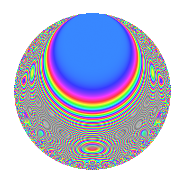# Properties

 Label 123.2.nLevel 123 Weight 2 Character orbit n Rep. character $$\chi_{123}(43,\cdot)$$ Character field $$\Q(\zeta_{20})$$ Dimension 48 Newforms 1 Sturm bound 28 Trace bound 0

# Related objects

## Defining parameters

 Level: $$N$$ = $$123 = 3 \cdot 41$$ Weight: $$k$$ = $$2$$ Character orbit: $$[\chi]$$ = 123.n (of order $$20$$ and degree $$8$$) Character conductor: $$\operatorname{cond}(\chi)$$ = $$41$$ Character field: $$\Q(\zeta_{20})$$ Newforms: $$1$$ Sturm bound: $$28$$ Trace bound: $$0$$

## Dimensions

The following table gives the dimensions of various subspaces of $$M_{2}(123, [\chi])$$.

Total New Old
Modular forms 128 48 80
Cusp forms 96 48 48
Eisenstein series 32 0 32

## Trace form

 $$48q + 8q^{4} + O(q^{10})$$ $$48q + 8q^{4} - 16q^{10} + 12q^{11} - 8q^{12} - 4q^{13} - 20q^{14} - 16q^{15} - 8q^{17} - 4q^{19} - 60q^{20} - 12q^{22} - 12q^{23} - 12q^{24} + 28q^{25} + 20q^{26} - 56q^{28} - 12q^{29} - 16q^{30} + 8q^{31} + 32q^{34} + 4q^{35} + 16q^{37} - 80q^{38} + 64q^{40} + 40q^{41} - 8q^{42} - 20q^{43} + 100q^{44} - 8q^{45} - 60q^{46} + 20q^{47} + 16q^{48} + 40q^{49} + 12q^{51} + 20q^{52} + 32q^{53} - 64q^{55} + 40q^{56} - 16q^{57} - 36q^{58} - 24q^{59} + 4q^{60} - 40q^{61} + 8q^{64} - 144q^{65} + 24q^{66} - 16q^{67} + 96q^{68} + 20q^{69} + 16q^{70} + 72q^{71} + 24q^{72} - 40q^{74} + 40q^{75} + 112q^{76} + 20q^{77} + 44q^{78} + 80q^{80} - 48q^{81} + 20q^{82} + 40q^{83} - 56q^{85} + 112q^{86} + 40q^{87} - 56q^{88} + 64q^{89} + 60q^{90} + 12q^{92} + 48q^{93} - 44q^{94} + 52q^{95} + 4q^{96} - 60q^{97} + 20q^{98} - 12q^{99} + O(q^{100})$$

## Decomposition of $$S_{2}^{\mathrm{new}}(123, [\chi])$$ into irreducible Hecke orbits

Label Dim. $$A$$ Field CM Traces $q$-expansion
$$a_2$$ $$a_3$$ $$a_5$$ $$a_7$$
123.2.n.a $$48$$ $$0.982$$ None $$0$$ $$0$$ $$0$$ $$0$$

## Decomposition of $$S_{2}^{\mathrm{old}}(123, [\chi])$$ into lower level spaces

$$S_{2}^{\mathrm{old}}(123, [\chi]) \cong$$ $$S_{2}^{\mathrm{new}}(41, [\chi])$$$$^{\oplus 2}$$